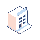• Bảng giá

# Change record color on Kanban view Odoo 14

I need help, I want to color the record in the CRM kanban view in red if my date field date_operation is less than current_date. I write this xml code but nothing happens.

N.B : I have created a date field current_date which gets date.today()

This is my code but the condition unable to executed

<xpath expr="//div[hasclass('oe_kanban_content')]" position="attributes">

<t t-if="record.date_operation.raw_value < record.current_date.raw_value">

<attribute name="t-attf-style">color: red;attribute>

t>

<t t-else="">

<attribute name="t-attf-style">color: blue;attribute>

t>

xpath>##### 2Câu trả lời

Thank you Mr. Jort de Vreeze for your solution, it work perfectly but the problem is in the computed field which calculated only one time, imagine that the date_deadline field is set for a later date, the expired field will not change in the future.

1Bình luậnSimply add the depends decorator to the compute method:

@api.depends('date_operation')
def _compute_expired(self):

If I understand you correctly, you could add a Boolean field in your model where you compute if the date is smaller than the current date:

```from datetime import datetime, timedelta

expired = fields.Boolean(    compute='_compute_expired',    string='Date expired?',    default=False,)def_compute_expired(self):    date_operation = datetime.now() - timedelta(days=1) # refer to your date_operation field            current_date = datetime.now()    self.expired = True if date_operation < current_date else False```

And then apply t-if for this field.